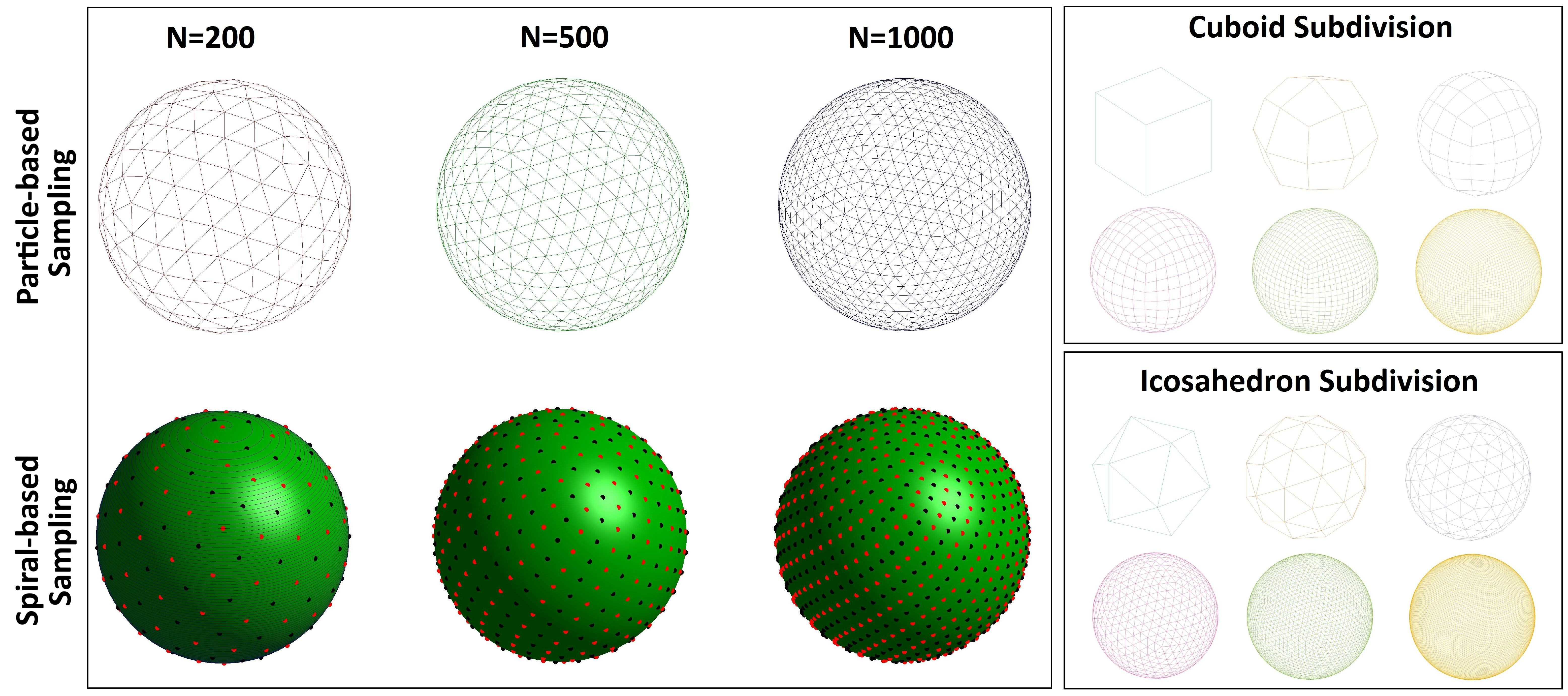## Suite of functions to perform uniform sampling of a sphere

version 1.6.0.1 (20.6 KB) by
Toolbox for generating uniform sampling patterns and decompositions of a unit sphere

Updated 04 Mar 2021

From GitHub

# S^2 Sampling ToolboxThe problem of finding a uniform distribution of points on a sphere has a relatively long history. Its emergence is commonly attributed to the physicist J. J. Thomson, who posed it in 1904 after creating his so-called plum pudding model of the atom . As such, the problem involves determination of a minimum energy configuration of N equally charged particles, confined to the surface of a sphere, that repel each other with a force given by Coulomb's law . Although the plum pudding model of the atom has long been dismissed, the original problem posed by Thomson has re-emerged across many areas of study and found practical applications in the fields as diverse as viral morphology, crystallography, physical chemistry, geophysics, acoustics, signal processing, computer graphics, and medical imaging (e.g., HARDI). The purpose of this submission is to provide Matlab users with a set of functions for generating uniform sampling patterns and decompositions of a unit sphere.## Summary of Main Functions

`ParticleSampleSphere.m`: generates an approximately uniform triangular tessellation of a unit sphere by using gradient descent to minimize a generalized electrostatic potential energy of a system of N charged particles. In this implementation, initial configuration of particles is based on random sampling of a sphere, but user-defined initializations are also permitted. This function can also be used to generate uniformly distributed sets of 2N particles comprised of N antipodal particle pairs. Since the optimization algorithm implemented in this function has O(N^2) complexity, it is not recommended that the function be used to optimize configurations of more than 1E3 particles (or particle pairs). Resolution of meshes obtained with this function can be increased to an arbitrary level with `SubdivideSphericalMesh.m`.

`SubdivideSphericalMesh.m`: increases resolution of triangular or quadrilateral spherical meshes. Given a base mesh, its resolution is increased by a sequence of k subdivisions. Suppose that No is the original number of mesh vertices, then the total number of vertices after k subdivisions will be Nk=4^k*(No – 2)+2. This relationship holds for both triangular and quadrilateral meshes.

`IcosahedronMesh.m`: generates triangular surface mesh of an icosahedron. High-quality spherical meshes can be easily obtained by subdividing this base mesh with the `SubdivideSphericalMesh.m` function.

`QuadCubeMesh.m`: generates quadrilateral mesh of a zero-centered unit cube. High-quality spherical meshes can be obtained by subdividing this base mesh with the `SubdivideSphericalMesh.m` function.

`SpiralSampleSphere.m`: generates N uniformly distributed point samples on a unit sphere using a spiral-based sampling method.

`RandSampleSphere.m`: performs uniform random or stratified random sampling of a unit sphere with N points.

## Demo 1: Uniformly Distributed Point Configurations on S^2 Via Charged Particle Sampling

``````% Uniformly distribute 200 charged particles across unit sphere
[V,Tri,~,Ue]=ParticleSampleSphere('N',200);

% Visualize optimization progress
figure('color','w')
plot(log10(1:numel(Ue)),Ue,'.-')
set(get(gca,'Title'),'String','Optimization Progress','FontSize',40)
set(gca,'FontSize',20,'XColor','k','YColor','k')
xlabel('log_{10}(Iteration #)','FontSize',30,'Color','k')
ylabel('Reisz s-Energy','FontSize',30,'Color','k')

% Visualize mesh based on computed configuration of particles
figure('color','w')
subplot(1,2,1)
fv=struct('faces',Tri,'vertices',V);
h=patch(fv);
set(h,'EdgeColor','b','FaceColor','w')
axis equal
hold on
plot3(V(:,1),V(:,2),V(:,3),'.k','MarkerSize',15)
set(gca,'XLim',[-1.1 1.1],'YLim',[-1.1 1.1],'ZLim',[-1.1 1.1])
view(3)
grid off
set(get(gca,'Title'),'String','N=200 (base mesh)','FontSize',30)

% Subdivide base mesh twice to obtain a spherical mesh of higher complexity
fv_new=SubdivideSphericalMesh(fv,2);
subplot(1,2,2)
h=patch(fv_new);
set(h,'EdgeColor','b','FaceColor','w')
axis equal
hold on
plot3(V(:,1),V(:,2),V(:,3),'.k','MarkerSize',15)
set(gca,'XLim',[-1.1 1.1],'YLim',[-1.1 1.1],'ZLim',[-1.1 1.1])
view(3)
grid off
set(get(gca,'Title'),'String','N=3170 (after 2 subdivisions)','FontSize',30)
``````

## Demo 2: Uniform Antipodally Symmetric Point Configurations on S^2 Via Charged Particle Sampling

``````% Uniformly distribute 100 antipodally symmetric particle pairs across unit sphere. Recall,
% an antipodal partner of particle P is -P (i.e., P reflected through the origin).
[V,Tri,~,Ue]=ParticleSampleSphere('N',100,'asym',true);

% Visualize optimization progress
figure('color','w')
subplot(1,2,1)
plot(log10(1:numel(Ue)),Ue,'.-')
set(get(gca,'Title'),'String','Optimization Progress','FontSize',40)
set(gca,'FontSize',20,'XColor','k','YColor','k')
xlabel('log_{10}(Iteration #)','FontSize',30,'Color','k')
ylabel('Reisz s-Energy','FontSize',30,'Color','k')

% Visualize mesh based on computed configuration of particles. Note that unlike the previous
% example, vertices of the mesh are (V;-V) and not (V). This is because -V are antipodal partners
% of V and must be combined with V to uniformly sample the entire sphere. However, just like in
% the previous example, computed mesh is also composed of 200 vertices, as there are 100 particle
% pairs.
subplot(1,2,2)
fv=struct('faces',Tri,'vertices',[V;-V]);
h=patch(fv);
set(h,'EdgeColor','b','FaceColor','w')
axis equal
set(gca,'XLim',[-1.1 1.1],'YLim',[-1.1 1.1],'ZLim',[-1.1 1.1])
view(3)
grid off
hold on
plot3(V(:,1),V(:,2),V(:,3),'.k','MarkerSize',15)
plot3(-V(:,1),-V(:,2),-V(:,3),'.r','MarkerSize',15)
set(get(gca,'Title'),'String','Final Mesh','FontSize',30)
``````

## Demo 3: Spiral-based S^2 Sampling

``````% Distribute 200 particles across unit sphere via spiral-based sampling method
[V,Tri]=SpiralSampleSphere(200,true);
``````

## Demo 4: Icosahedron-based S^2 Decomposition

``````% Get base icosahedron mesh
TR=IcosahedronMesh; % also try this with `DodecahedronMesh.m`

% Subdivide base mesh and visualize the results
figure('color','w')
ha=subplot(2,3,1);
h=trimesh(TR); set(h,'EdgeColor','b','FaceColor','w')
axis equal
set(get(ha,'Title'),'String','base mesh (N=12)')
for i=2:6
ha=subplot(2,3,i);
TR=SubdivideSphericalMesh(TR,1);
h=trimesh(TR); set(h,'EdgeColor','b','FaceColor','w')
axis equal
set(get(ha,'Title'),'String',sprintf('N=%u',4^(i-1)*10+2))
drawnow
end
``````

## Demo 5: Cuboid-based S^2 Decomposition

``````% Get quad mesh of a unit cube

% Subdivide base mesh and visualize the results
figure('color','w')
ha=subplot(2,3,1);
h=patch(fv); set(h,'EdgeColor','b','FaceColor','w')
view(3)
grid on
axis equal
set(gca,'XLim',[-1.1 1.1],'YLim',[-1.1 1.1],'ZLim',[-1.1 1.1])
set(get(ha,'Title'),'String','base mesh (N=8)')
for i=2:6
ha=subplot(2,3,i);
fv=SubdivideSphericalMesh(fv,1);
h=patch(fv); set(h,'EdgeColor','b','FaceColor','w')
axis equal
view(3)
grid on
set(gca,'XLim',[-1.1 1.1],'YLim',[-1.1 1.1],'ZLim',[-1.1 1.1])
set(get(ha,'Title'),'String',sprintf('N=%u',4^(i-1)*6+2))
drawnow
end
``````

MIT © 2019 Anton Semechko (a.semechko@gmail.com)

### Cite As

Anton Semechko (2021). Suite of functions to perform uniform sampling of a sphere (https://github.com/AntonSemechko/S2-Sampling-Toolbox), GitHub. Retrieved .

##### MATLAB Release Compatibility
Created with R2011b
Compatible with any release
##### Platform Compatibility
Windows macOS Linux